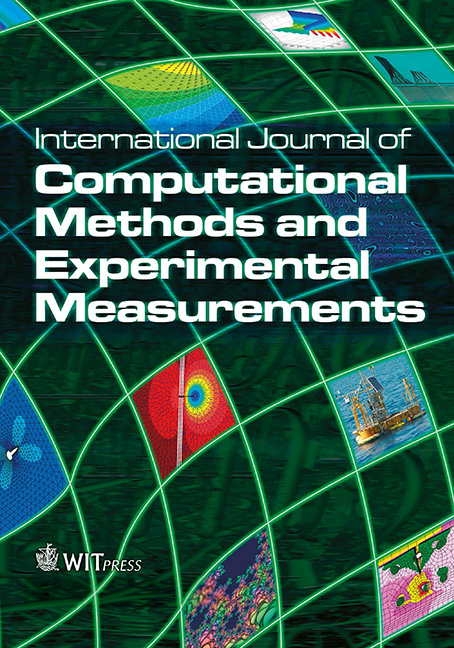WIT Press

SCATTERING OF WATER WAVES BY A POROUS CIRCULAR ARC-SHAPED BARRIER SUBMERGED IN OCEAN

Price

Free (open access)Volume

Volume 4 (2016), Issue 4

Pages

10

Page Range

532 - 542

Paper DOI

10.2495/CMEM-V4-N4-532-542

WIT Press

Author(s)

DIBAKAR MONDAL & SUDESHNA BANERJEA

Abstract

In this paper, we study the problem of scattering of surface water waves by a thin circular arc shaped porous plate submerged in the deep ocean. The problem is formulated in terms of a hypersingular integral equation of the second kind in terms of an unknown function representing the difference of potential function across the curved barrier. The hypersingular integral equation is then solved by a collocation method after expanding the unknown function in terms of Chebyshev polynomials of the second kind. Using the solution of the hyper-singular integral equation, the reflection coefficient, transmission coefficient and energy dissipation coefficient are computed and depicted graphically against the wave number. Known results for the rigid curved barrier are recovered. It is observed that the porosity of the barrier reduces the reflection and transmission of the waves and enhances the dissipation of wave energy. The reflection coefficient and dissipation of wave energy decreases as the length of the porous curved barrier increases. Also the reflection coefficient is almost independent of the inertial force coefficient of the material of the porous barrier. However, the inertial force coefficient of the material of the porous barrier enhances transmission and reduces dissipation of wave energy.

Keywords

curved porous plate, dissipation of wave energy, reflection coefficient, transmission coefficient, water wave scattering